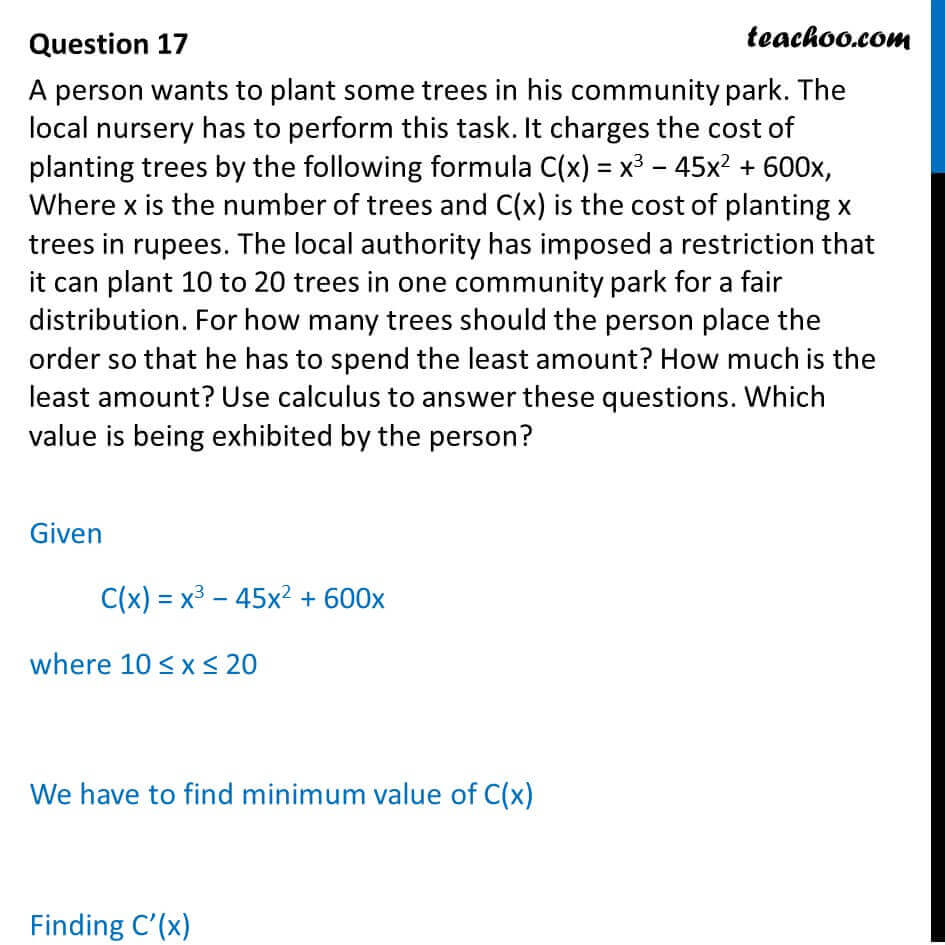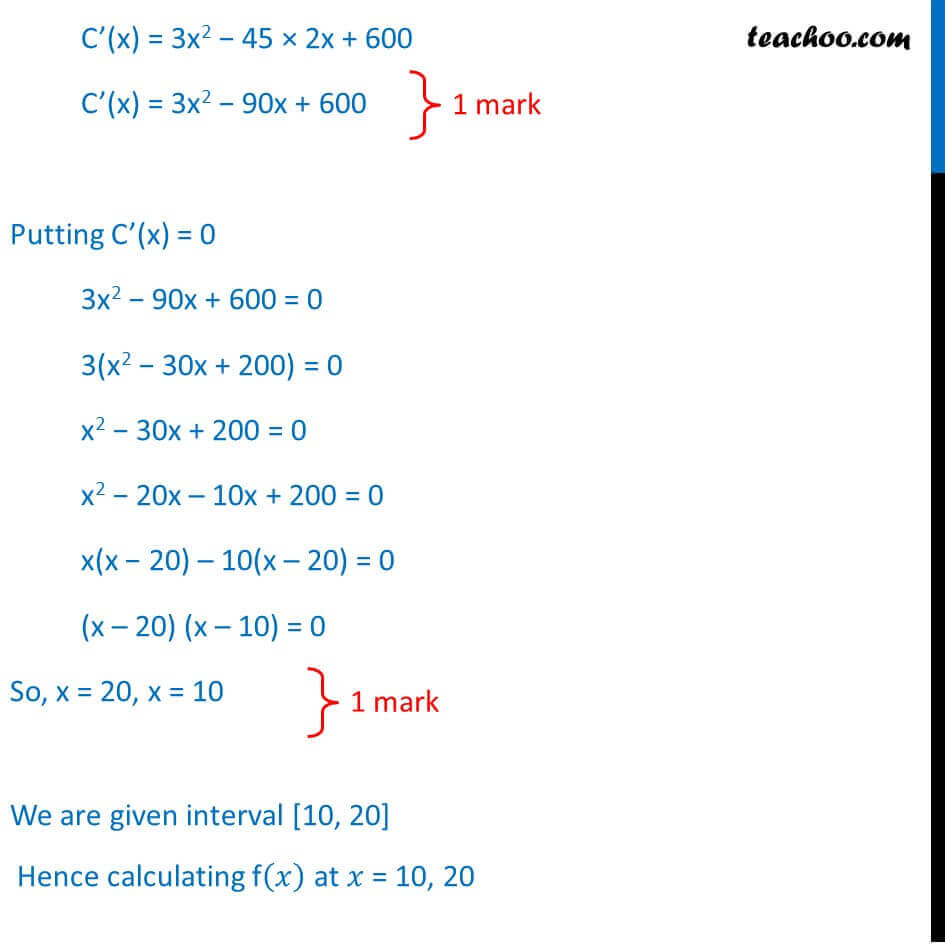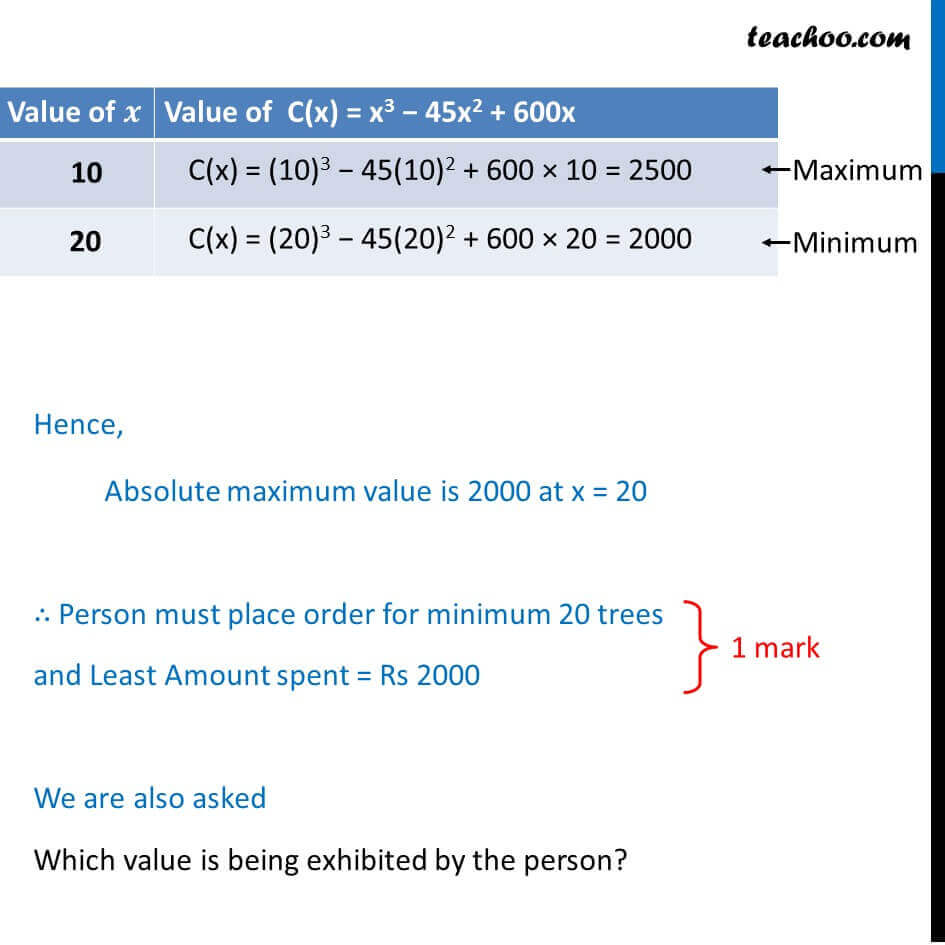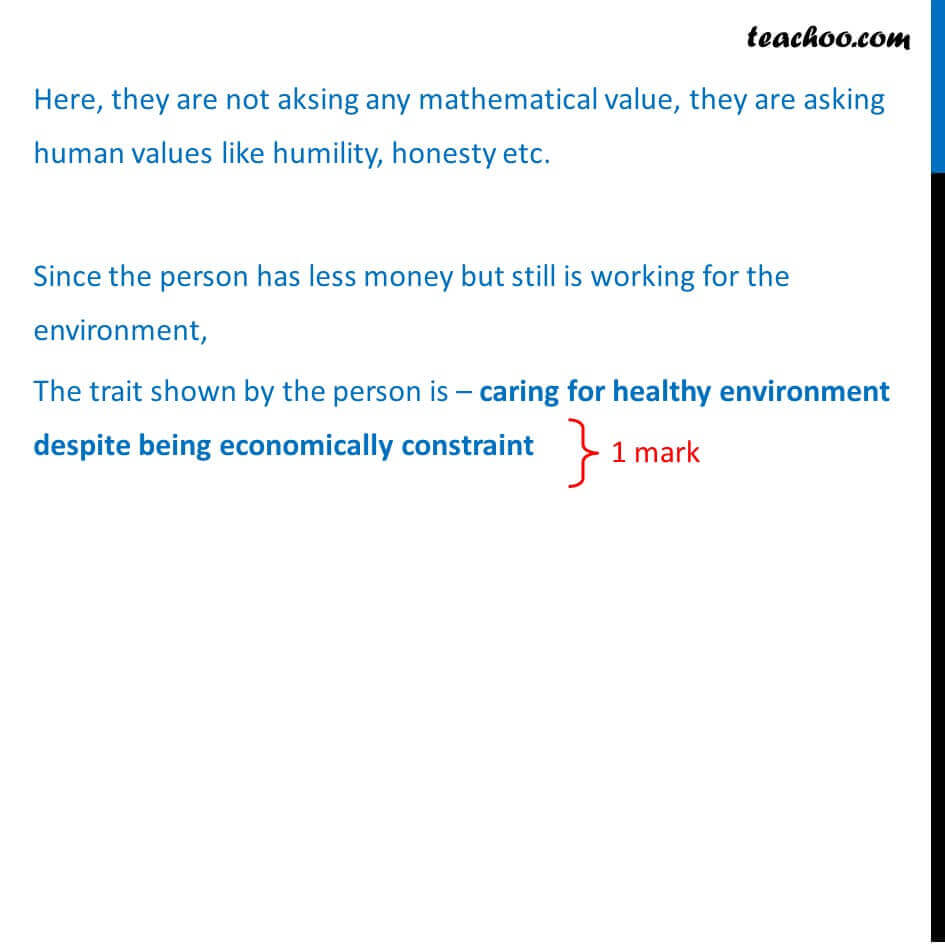CBSE Class 12 Sample Paper for 2018 Boards

Class 12
Solutions of Sample Papers and Past Year Papers - for Class 12 Boards

### A person wants to plant some trees in his community park. The local nursery has to perform this task. It charges the cost of planting trees by the following formula C(x) = x 3 − 45x 2 + 600x, Where x is the number of trees and C(x) is the cost of planting x trees in rupees. The local authority has imposed a restriction that it can plant 10 to 20 trees in one community park for a fair distribution. For how many trees should the person place the order so that he has to spend the least amount? How much is the least amount? Use calculus to answer these questions. Which value is being exhibited by the person?

This is a question of CBSE Sample Paper - Class 12 - 2017/18.Learn in your speed, with individual attention - Teachoo Maths 1-on-1 Class

### Transcript

Question 17 A person wants to plant some trees in his community park. The local nursery has to perform this task. It charges the cost of planting trees by the following formula C(x) = x3 45x2 + 600x, Where x is the number of trees and C(x) is the cost of planting x trees in rupees. The local authority has imposed a restriction that it can plant 10 to 20 trees in one community park for a fair distribution. For how many trees should the person place the order so that he has to spend the least amount? How much is the least amount? Use calculus to answer these questions. Which value is being exhibited by the person? Given C(x) = x3 45x2 + 600x where 10 x 20 We have to find minimum value of C(x) Finding C (x) C (x) = 3x2 45 2x + 600 C (x) = 3x2 90x + 600 Putting C (x) = 0 3x2 90x + 600 = 0 3(x2 30x + 200) = 0 x2 30x + 200 = 0 x2 20x 10x + 200 = 0 x(x 20) 10(x 20) = 0 (x 20) (x 10) = 0 So, x = 20, x = 10 We are given interval [10, 20] Hence calculating f( ) at = 10, 20 Hence, Absolute maximum value is 2000 at x = 20 Person must place order for minimum 20 trees and Least Amount spent = Rs 2000 We are also asked Which value is being exhibited by the person? Here, they are not aksing any mathematical value, they are asking human values like humility, honesty etc. Since the person has less money but still is working for the environment, The trait shown by the person is caring for healthy environment despite being economically constraint